# An Introduction to Particle Characterization

Size and shape are the features that most impact particulate substance behavior. Spherical beads are easy to characterize by their diameter, a single size measure.

More irregular shapes are harder to be characterized, but for powders of use in manufacturing it is a requirement to determine a small set of numbers that describe the sample in its entirety and that can predict performance. The types of shapes that are contained in the sample will indicate what size and shape parameters are required.

As there are a big amount of particles, size and shape data are statistical. Histograms are the optimal method to demonstrate statistical distributions of a measure or variable, and there are varied means and measures of spread to characterize a distribution with the use of only a few numbers.

## Distribution Histograms

To illustrate the sizes that exist in a sample, the size range is split into small size classes or “bins”, and the number of particles contained in each size bin are counted. The following graph is a size histogram and it shows a good view of the actual distribution. Size data is usually depicted graphically on a log scale axis, to improve how the small sizes are shown.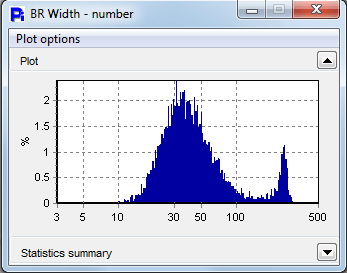Typical size data histogram

Non-size data such as smoothness, circularity or aspect ratio is demonstrated on a linear scale: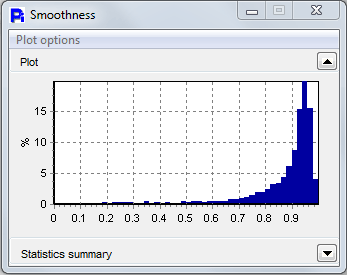Typical linear axis histogram

The more fine the division of the axis, the more precise the means and distribution shape figures that are drawn from the histogram will be. If too finely divided, bins with no counts may occur, and these will appear as gaps in the graph, particularly at the low end on a log axis. In the Particle Insight, the number of divisions and the size range are settable in Analysis Conditions.

A volume-weighted histogram plots the relative volumes contained in the size classes: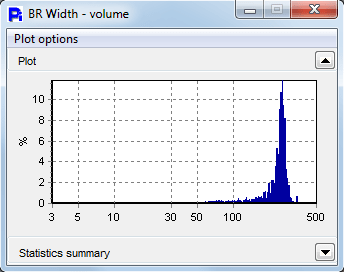A volume-weighted histogram

The distribution of the volume can only be calculated precisely if particles are round or if there is accurate information regarding shape. Inferring a volume distribution from single-size measure counts will not be correct if particles are not spherical.

Surface area weighting can be carried out in a similar manner and with the same considerations. On a log scale, a number (unweighted) plot stresses the small sizes, while volume weighting stresses the big sizes and may not illustrate the smallest sizes at all. Weighting by surface area will be between number and volume.

The following chart shows how the number and volume weighting differs.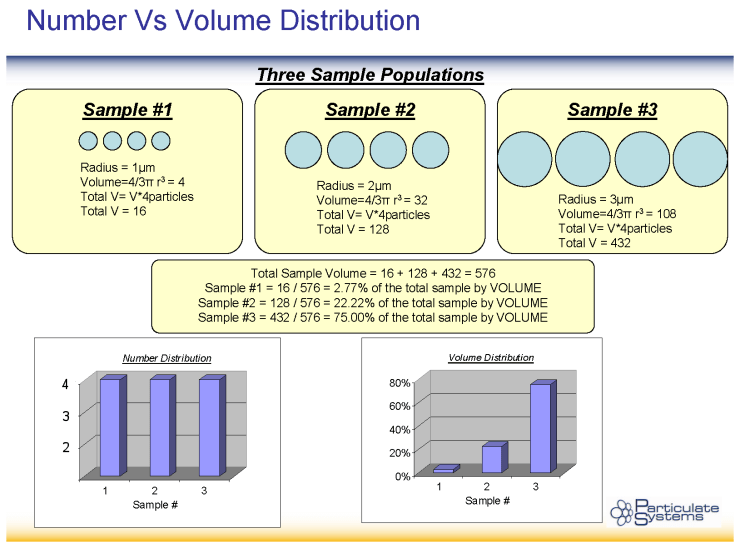Difference between number and volume weighting

The graphs become more smooth if the particle count is 10,000 or above. Even if the count is high, if there are extremely small sizes within the sample, occasionally some size classes or “bins” near the bottom end will appear that do not demonstrate any counts, as seen in the following plot :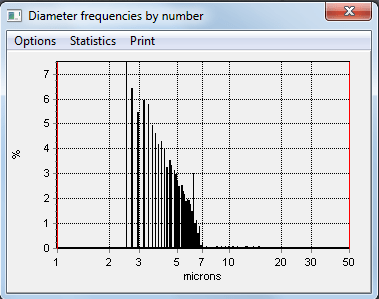Empty bins near low end

This occurs due to particle areas that are determined as an integer number of pixels, and the micron equivalents of 3 and 4 pixels, as an example, may not land in consecutive bins. More than one size bins may be skipped. This effect is seen predominantly with cameras of a lower resolution (1 megapixel or less). It is also impacted by the calibration of the size.

The volume-weighted histogram frequently demonstrates isolated counts at the high end, as in the below:Isolated spikes near high end

This occurs because a single extremely big particle can be indicative of an important portion of the complete volume, and thus on the volume-weighted histogram that single count will stand out. Camera resolution is not a contributor to this effect.

## Characterization of Distributions

### Means

A distribution is characterized by a mean as a single number. A mean value allows some information of the sizes present but does not issue any indication about the shape of the distribution or how broad or narrow it is. The number or arithmetic mean is purely the average value. It is frequently denoted as D1,0. Other means take into account volume weighting and area.

Assuming sphericity of particles, the generic definition of a weighted Dp,q mean diameter is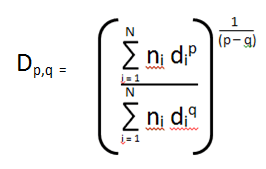Definition of Dp,q means

The diameter means that are most often of use in characterizing a particle sample are: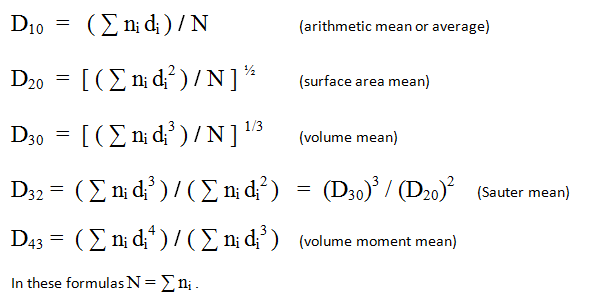In these definitions, ni is the count in size bin number i, and di is the representative diameter of that size bin. The sums are over all particles, and N is the particle count total.

Descriptions of these means in words are as follows:

Arithmetic mean diameter, D[1,0] : the average of the diameters of all the particles in the sample.

Surface mean diameter, D[2,0] : the diameter of a particle whose surface area, if multiplied by the total number of droplets, will equal the total surface area of the sample.

Volume mean diameter, D[3,0] : the diameter of a particle whose volume, if multiplied by the total number of particles, will equate all of the sample’s volume.

Surface moment mean diameter, D[3,2] (“Sauter mean”): the diameter of a particle whose ratio of volume to surface area is the same as that of the complete sample. Mathematically, if V is the total volume and A is the total surface area of the sample, D3,2 = 6 * (V / A).

Volume moment mean diameter, D[4,3]. This value is a superior indicator than other means of which particle sizes contain the majority of the volume.

The Mode is the most frequent size present.

The Harmonic mean is N / Σ (ni / di)

### Geometric Means

Geometric means reflect the visual weighting of a log-size axis. The geometric mean diameter will appear as the center of a distribution on a log scale, while the usual arithmetic mean may sit a lot lower on the size scale (as smaller sizes are a lot more numerous than larger sizes).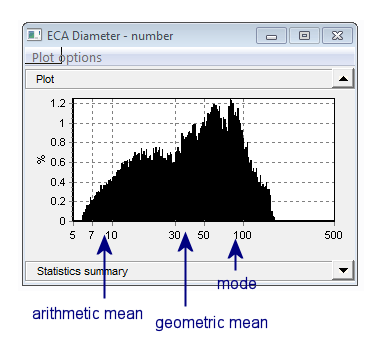Arithmetic and geometric means

To compute the geometric mean, use the logs of the x-axis values ( log (di) in place of di ) :

The Geometric mean is  Σ [ni  log(di)] / N

With the use of the same manner, the geometric versions of the other means and standard deviation may be calculated.

Standard deviation measures how wide the distribution is: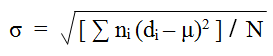where μ = mean diameter (D10). It has units of microns (for a size distribution).

The Coefficient of Variance is the ratio of the standard deviation to the mean: CV = σ / μ . To express as a percent multiply by 100. Being a ratio, this statistic does not have units.

### Percentiles

Percentiles are a way of conducting size information as one or more numbers. The Median size splits the particles into two parts containing equal counts. Strictly speaking, this is the Number Median. It is also known as the 50th or the 50% percentile.

The 10th percentile defines the size with the property that 10% of the particle count is less than that size. Any other percentile can be defined similarly. Commonly, three percentiles (10%, 50% and 90%) are used as a characterization that is uncomplicated but provides additional information to a single mean.

The Volume Median, or 50th percentile by volume, splits the volume of the sample into two equal pieces. The two classes will hold equal volume but not an equal count of particles. Other volume percentiles are defined similarly; as an example, the 25th volume percentile is the size such that particles smaller than that size are representative of a quarter of the volume.

### Other Characterizations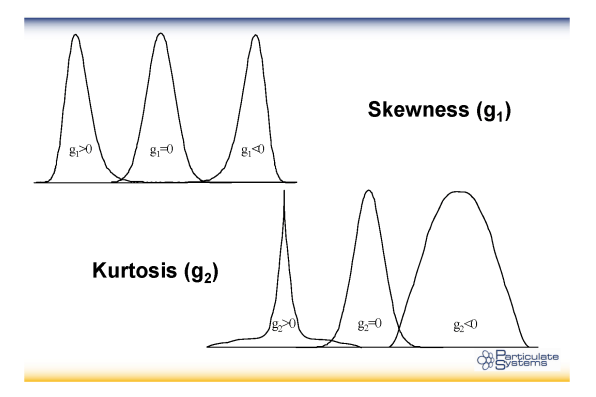Skewness is an indicator of how asymmetrical the shape of the distribution is, about the center. A positive value will mean there are further counts on the right side of center, normally in the appearance of a tail. A negative value will mean it tails to the left.

Skewness = Σ ni  (di – μ)3 / (σ3 N)  (σ = std. dev., μ = mean)

Kurtosis is an indicator of how much the shape differs from the typical bell curve in a vertical sense.

Kurtosis = [Σ ni  (di – μ)4 / (σ4 N)] / - 3  (σ = std. dev., μ = mean)This information has been sourced, reviewed and adapted from materials provided by Vision Analytical Inc.

## Citations

• APA

Vision Analytical Inc.. (2019, November 05). An Introduction to Particle Characterization. AZoM. Retrieved on July 06, 2020 from https://www.azom.com/article.aspx?ArticleID=18502.

• MLA

Vision Analytical Inc.. "An Introduction to Particle Characterization". AZoM. 06 July 2020. <https://www.azom.com/article.aspx?ArticleID=18502>.

• Chicago

Vision Analytical Inc.. "An Introduction to Particle Characterization". AZoM. https://www.azom.com/article.aspx?ArticleID=18502. (accessed July 06, 2020).

• Harvard

Vision Analytical Inc.. 2019. An Introduction to Particle Characterization. AZoM, viewed 06 July 2020, https://www.azom.com/article.aspx?ArticleID=18502.

## Tell Us What You Think